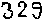# PkANN

## PkANN v1.0

```Last Modified: 10 December 2013

Shankar Agarwal (shankar.agarwal@obspm.fr)
Filipe Abdalla (fba@star.ucl.ac.uk)
Hume Feldman (feldman@ku.edu)
Ofer Lahav (lahav@star.ucl.ac.uk)
```

```By downloading and using the PkANN code, you implicitly agree to:
Acknowledgement the PkANN developers and cite the PkANN-II paper (http://arxiv.org/abs/1312.2101).
```

## PkANN CONTENTS

```README.html
make.sh
unix.sh
H0ANN/
pkANN/
Testing_set/
```

## PURPOSE

PkANN code interpolates the non-linear matter power spectrum, P(k). PkANN can handle spatially flat cosmological models with cold dark matter + massive neutrinos + dark energy with constant equation of state w (flat wCDM + massive neutrinos). This code forms the basis of the two papers: PkANN-I (http://arxiv.org/abs/1203.1695) and PkANN-II (http://arxiv.org/abs/1312.2101). PkANN-I, using Halofit spectra as mock N-body simulations, shows the feasibility of using Artificial Neural Networks (ANNs) for interpolating the non-linear matter power spectrum at per cent level accuracy. PkANN-II describes the construction of a committee of 16 ANNs using a suite of 6380 N-body simulations spanning 580 cosmologies.

```PkANN is valid (for all redshifts less than or equal to 2) for cosmological models within the following range:
0.110  < Omega_m h^2 < 0.165   physical matter density (cold dark matter + baryons + massive neutrinos)
0.021  < omega_b h^2 < 0.024   physical baryon density
0.85   < n_s         < 1.05    primordial scalar spectral index
-1.35  < w           < -0.65   constant equation of state parameter for dark energy
0.60   < sigma_8     < 0.95    amplitude of fluctuation on an 8 Mpc/h scale
0      < mass_nu     < 1.1 eV  total neutrino mass (3 degenerate species)

To run the code (explained later), user must input the above six cosmological parameters and the redshift at which to compute the spectrum.
The Hubble parameter, h, is NOT input by the user. h is computed by H0ANN code using WMAP 7-year + BAO contraint on the acoustic scale.
The procedure used by H0ANN code to compute h is outlined in Section 3.1, http://arxiv.org/abs/1203.1695.
```

```PkANN code is publicly available at http://zuserver2.star.ucl.ac.uk/~fba/PkANN/PkANN.tar.gz

If you have wget installed on your system, you can download PkANN using the following Shell command:

% wget http://zuserver2.star.ucl.ac.uk/~fba/PkANN/PkANN.tar.gz

NOTE: % designates your command line prompt.
```

## COMPILE

```You will need Fortran 90 (or higher) and C++ compilers. To compile the code, do the following:

% tar -xvzf PkANN.tar.gz
% cd PkANN
% ./make.sh

This will compile two programs: H0ANN, pkANN.
The purpose of these programs is explained later in the section: PROGRAM DETAILS.

NOTE: In case of compilation errors, edit the Makefiles inside H0ANN/, H0ANN/cosmo/ and pkANN/ directories.
```

## RUN

```To run the code, do the following:

1. Edit Testing_set/parameters.txt to enter the desired cosmological model. Each line is a unique cosmology and specified by six values,
corresponding to the six parameters discussed above (in the same sequence). For each cosmology, enter its parameters on a new line.
NOTE: We have supplied a sample Testing_set/parameters.txt file with 10 cosmological models.

2. Edit Testing_set/z_list.txt to enter the redshifts (eg. 1.83, 0, 0.947, 2, 0.5) at which you want to evaluate the non-linear matter
power spectrum. You can enter as many redshift values as you want (each value on a new line).
NOTE: PkANN code is valid for all redshifts less than or equal to 2.

3. Run unix.sh as "./unix.sh"

The non-linear matter power spectra for your selected cosmologies get generated in the Testing_set/PkANN_predictions/ subdirectory.
The Hubble parameter for your selected cosmologies can be obtained from Testing_set/models_parameters.txt file.

NOTE: The format of all output files is described in the section: PROGRAM DETAILS.
```

## PROGRAM DETAILS

```1. H0ANN:  The purpose of this program is to predict the Hubble parameter h (for each cosmology in Testing_set/parameters.txt file)
that best fits the CMB constraint (d_lss=302.54*r_s/pi), where
d_lss = Comoving distance to the last scattering surface, and
r_s   = Comoving sound horizon at the redshift of last scattering, z_lss.

This program reads the cosmological models from Testing_set/parameters.txt file. It writes the Hubble parameter in a
temporary file hubble.txt in H0ANN/cosmo/ subdirectory. The file H0ANN/cosmo/cosmo.f90 reads hubble.txt and computes
the normalized density parameters (Omega_baryon, Omega_cdm etc.), z_lss, r_s (in Mpc/h) and the comoving distance to
last scattering (d_ls, in Mpc/h). All these parameters, along with h, and your chosen values of spectral index (n_s),
dark energy equation of state (w) and sigma_8, are written in Testing_set/models_parameters.txt file. The last column
is the difference (d_ls-d_lss) between the distance to last scattering surface (d_ls) computed using H0ANN's prediction
of h, and the distance to last scattering surface (d_lss) computed using d_lss=302.54*r_s/pi. The difference is a measure
of how accurately H0ANN predicts the CMB-constrained Hubble parameter.

The input/output is automatically performed by H0ANN program. Manual intervention is not needed.

2. pkANN:  The purpose of this program is to predict the non-linear matter power spectrum for all cosmological models (in
Testing_set/parameters.txt file), at all redshift values (in Testing_set/z_list.txt file).

This program reads the cosmological models (from Testing_set/parameters.txt file) and the redshift values
(from Testing_set/z_list.txt file). The output files of this program are:

(a) Testing_set/PkANN_predictions/model_**/ps_nl_*.dat: model_** are the cosmological models model_1, model_2 ... etc.
ps_nl_*.dat are the non-linear matter power spectrum files (one for each redshift). Each file has two columns:
k(h/Mpc), non-linear matter power spectrum (Mpc/h)^3. NOTE: These files are numbered 1,2,3,4 ... etc. in the same
order as the redshift values in Testing_set/z_list.txt file.

The input/output is automatically performed by pkANN program. Manual intervention is not needed.```